Welcome to Study Material Solution
Leave Comment

## INTRODUCTION

We use force all the time. We use force to open a door, to pick up the school bag,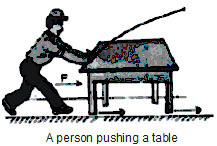to brush our teeth, to squeeze out toothpaste from a tube, to turn on a tap and so on. Even the earth is exerting a force on us all time. It is pulling all of us and all things on earth towards it.In fact we use force for every single action of ours! You have studied about force in previous classes. Do you remember what a force is ?

FORCE

The external agent which tends to set a body in motion or which changes the speed and direction of motion of a body or which can change the shape of a body is called force.
(i) To push or to pull is also called as force.
(ii) It is a vector quantity

UNIT OF FORCE

(i) The SI unit (in standard international system) of force is called Newton and its symbol is N (kg × m/sec2)
(ii) C.G.S unit of force is dynes (gm cm/s2)
1 Newton = 105 dynes,
1 N     = kg – m/s2
= 1(1000 gm) (100 cm/s2)
= 105 gm–cm/s2
So that 1 N = 105 dyne

EFFECT OF FORCE

1. Force can move a body lying at rest
Example :(a) Kicking a stationary football.           (b)     Lifting a book kept on a table top.
(c) Hitting a stationary ball with a bat.

2. FORCE CAN STOP A MOVING BODY
Example :
(a) A goalkeeper stopping a football moving towards the goal post.
(b) A moving bicycle coming to rest on applying brakes. Here the frictional force between the brake lining of the brake shoe and the bicycle wheel rim, acts to stop the bicycle.

3.FORCE CAN CHANGE THE SPEED OF A MOVING BODY
Ex. To decrease speed, force is applied in a direction which is opposite to the motion of the body. If we apply force in the direction of motion of the body, it
tends to increase the speed of the body. For example, if your friend is riding a bicycle and you push the bicycle in the same direction. The speed of bicycle will
increase. On the other hand if you pull the bicycle, i.e., you apply force against the direction of motion, the speed of bicycle decreases.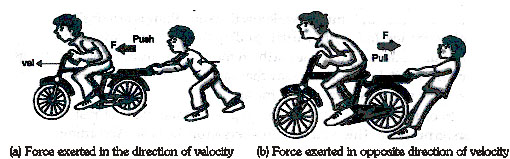4. FORCE CAN CHANGE THE DIRECTION OF MOVING BODY
Example :
(a) A carrom counter changes its direction after a collision.
(b) When a batsman hits a ball, he changes the direction of the ball.
(c) When a stone is rotated in a circular path, the direction of motion of the stone change continuously. The force acting on the stone towards the centre
of the circular path is responsible for changing the direction of the stone.
(d) A football player hitting a ball coming towards him, towards the goal post.

5.   FORCE CAN CHANGE THE SHAPE AND SIZE OF AN OBJECT
Example
(a) When we squeeze a toothpaste tube, it gets flattened.
(b) When we stretch a rubber band, its shape and size changes.
(c) On stretching a spring, its length changes.

6.    FORCE CAN MAKE A BODY ROTATE
Example :
(a) When electric current is passed through a motor of a ceiling fan, forces are produced in the dynamo (or motor) which makes the fan to rotate.
(b) For closing a door you apply a push.
To conclude --> a force can accelerate a body, change its shape and size or can produce a rotational effect.

TYPES OF FORCES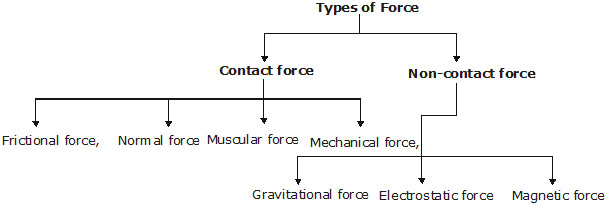(A) CONTACT FORCE  :  When a force involves direct contact of two bodies we call the force a contact force.
(i) FRICTIONAL FORCE :– The force which acts to reduce relative motion between the surfaces of contact is called the frictional force.
Suppose a block is moving on a horizontal surface with a velocity u. The darkened line at the bottom of the block is the surface of contact of the block with floor.
We notice that this surface of contact is moving towards right with respect to the horizontal surface. To reducing this relative motion, frictional force acts. Frictional
force is exerted by the floor on the block in a direction opposite to velocity.Note :  Friction force :    (i)    Frictional force acts in a direction opposite to that of the motion
(ii)      The smoother the surface the lesser is the frictional force.
(a) Static friction : Which acts when a body is stationary.
(b) Kinetic friction : Which acts when a body is moving.

(ii) NORMAL FORCE : The force acting on a body perpendicular to the surface of contact is called a normal force.
Note : Tension and spring force are also contact forces.
(iii) MUSCULAR FORCE : The force resulting due to the action of muscles is known as the muscular force. Animals also make use of muscular force to carry
out their physical activities and other tasks. Animals like bullocks, horses, donkey and camels are used to perform various tasks for us. In performing these tasks they use
muscular force. Since muscular force can be applied only when it is in contact with an object. This force is also known as Biological force.
(iv) MECHANICAL FORCE : The force exerted by a machine is called mechanical force. Machines do not produce force by themselves. In order to produce force they
need energy from other sources. Mechanical force produced by a car engine, and mechanical force produced by the turbines in a hydroelectric power station are examples of Mechanical force.

(B) NON-CONTACT FORCE
The force which a body applies on another body when the two bodies are not in contact with each other is called non-contact force.
(i) GRAVITATIONAL FORCE : The force of attraction which one body exerts on the other because of their masses is called gravitational force.
Gravitational force is the weakest force in nature and still this force is responsible for the revolution of earth around the sun.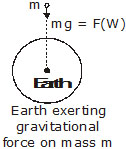Force of gravity on a body is equal to the true weight of the body.
The force with which earth attracts a body is called the force of gravity. A body of mass m is attracted by earth towards its centre. This force is also called the weight (W) of the body.

W=mg

(ii)  ECLECTROSTATIC FORCE : The force which results due to the repulsion of similar charges or attraction of opposite charges is called electrostatic
force. If we rub a plastic object like a pen or a comb with hair and bring it close to tiny bits of paper, the bits of paper get attracted to the plastic object. This is due
to electric force. Tiny particles of dust and smoke can also be attracted by electrostatic force. This method is used in air purifiers and in factories to purify air in chimneys,
before letting it escape into the atmosphere.
(iii) MAGNETIC FORCE :  This is the force exerted by magnets on each other and on some metals like iron and nickel. Since magnets attract iron, magnets are
used to separate waste iron object form garbage dumps so that they can be recycled. Magnetic force and electrostatic force are inter-related and are together
called electro-magnetic force.

RESULTANT FORCE
If a single force acting on a body produces the same acceleration as produced by a number of forces, then single force is called the resultant of these individual forces.
The resultant force is also called the net force.
Ex.    Suppose an almirah is being pushed by two persons A and B. Let the force applied by A be F1 = 2N and that by B be F2 = 3N. These two forces together
will result in some displacement of the almirah in a given time. In another situation, a person C is pushing the same almirah kept initially in the position as that
of the previous situation. The person C is applying a force F = 5N in the same direction as that of A and B.

BALANCED FORCES
When the force acting on a body cancel out the effects of each other in such a way that the resulting force is zero, then these forces are said to be balanced.
Ex.    Let us consider a rigid almirah kept at rest on a horizontal surface. Let two forces F1 and F2 act simultaneously on the almirah from opposite directions. If the two
forces are equal, the effects produced by one force get cancelled by the effect produced by the other. The net force or the resultant force is then zero. The almirah continues
to remain at rest. When a numbers of forces acting on a body do not cause any change in its state of rest, or of uniform motion is a straight line then the force are said to be balanced forces.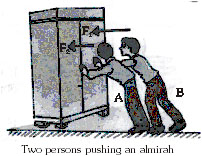UNBALANCED FORCES
If number of forces acting on a body                produce an acceleration in the body, then the forces acting are called unbalanced.
Ex.    Suppose F1 = 5 Newton and F2 = 3 Newton. In this case a resultant force is acting on the almirah towards the direction of F1. We say that an unbalanced
force of 2 Newton will act on the almirah towards the direction of F1.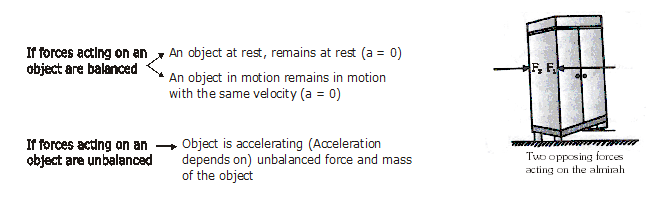NEWTON'S LAWS OF MOTION
(A) Newton´s I law : A body cannot change its state of motion by itself. If the object is at rest it will remain at rest and if it is in uniform motion, it continues to be
in motion unless some external force is applied on it.

Inertia :
(i) There is an inherent property of an object by virtue of which it cannot change its state of motion or rest by if self. This property is called 'inertia'.
(ii) Inertia is of two types– inertia of rest and inertia of motion.

(a) Inertia of rest: If the body is at rest, it will continue to be at rest unless some external force is applied on it. Examples are following.
(i) When a train at rest starts moving suddenly, a passenger standing inside the compartment tends to fall backward.
(ii) When a carpet is beaten up with a stick, the dust particles are detached.
(iii) When a bullet is fired into a glass pane, it pierces a hole only at the pt where the bullet hits the glass without breaking the entire glass pane into pieces.
(b) Inertia of motion : When a body is in uniform motion, it will continue to remain in its uniform motion, i.e. it resists any change in its state of motion due to inertia of motion.
(i) When a person jumps out of a moving bus, he should run in the direction in which bus is moving otherwise he will fall down.
(ii)  A train moving with a uniform speed and if a ball is thrown upwards inside the train by a passenger, then the ball comes back to his hand.

Mass and Inertia :
(i) Larger the mass of the body, larger is the inertia.
eg. it is more difficult to stop a cricket ball than a tennis ball.

(B) Newton's second law of motion
Force F is equal to the product of mass, m of a body and acceleration, a produced in the body due to that force. i.e. F = ma
Acceleration: Acceleration is the rate of change of velocity.Note: Newton's first law of motion gives a qualitative idea of force, while the second law provides us an idea to measure the force.

(C) Newton's third law of motion
(i) Newton's third law of motion states that " if a body A exerts a force on the body B, the body B will also exert an equal and opposite force on A."
(ii) The force exerted by A on B is called action while the force exerted by B on A is called the reaction.
(iii) Newton's third law is also stated as "to every action there is an equal and opposite reaction."
(iv)  Action and reaction always act on different bodies.

Applications of Newton's III law :
(i) Recoil of a gun : where the bullet is fired from a gun, an equal and opposite force is applied on the gun, due to which the gun recoils in backward direction.
(ii) Application in walking : while moving in forward direction we push the ground backwards that is the action. An equal and opposite force is applied by the
ground on the man, thus the reaction due to which man moves forward.
(iii) Rowing a boat in river : when we push the water backward with the help of oars (applying a force backward), an equal and opposite force acts on the boat. This is
the reaction which moves the boat forward.
(iv)Launching Rocket : In rocket, gases are produced in large amount. Due to internal combustion they come out and move backwards with an equal and opposite force
which in turn acts on the rocket and moves it forward.

MASS & WEIGHT
MASS The amount of matter contained in a body is called its mass
OR
The measure of the quantity of matter in a body is called its mass.
The mass of a body is a scalar quantity. It is independent of surroundings and the position of the body. It is a constant quantity for a given body.
Mass is measured in kilogram (kg) in S.I. System.
WEIGHT
Everybody on the surface of earth is attracted towards the centre of the earth. The force of attraction  depends upon the mass of the body and the acceleration due
to gravity. The weight of the body is the force with which it is attracted towards the centre of the earth. We know
F = ma
The acceleration produced by the force of attraction of the earth is known as acceleration due to gravity i.e., g
\    F = ma = mg
But by definition this force is equal to the weight of the body i.e., F = W.
\    W = mg
SI unit of weight is Newton (N) and in CGS, it is measured in dyne.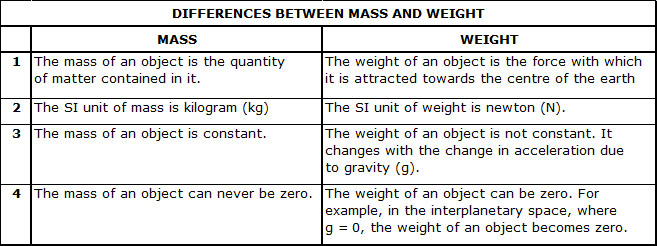IMPORTANT POINTS
(i) Mathematical Representation of force : Mathematically, force F is equal to the product of mass M of a body and acceleration, a produced in the body due to that force. i.e. F = ma
Acceleration : Mostly the velocity of a moving object changes either in magnitude or in direction or in both when the object moves. The body is then said to have
acceleration. So it is the rate of change of velocity i.e. change in velocity in unit time is said to be acceleration. ( it is vector quantity)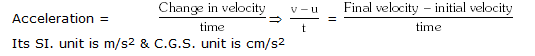Its SI. unit is m/s2 & C.G.S. unit is cm/s2
(ii) Gravitational force : According to Newton
"Every body in the universe attract another body with a force which is directly proportional to product of their masses and inversely proportional to square of the distance between them."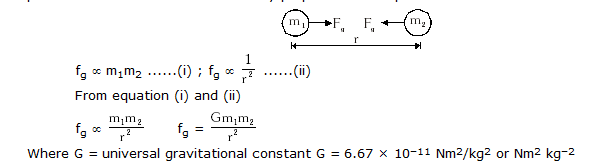Where G = universal gravitational constant G = 6.67 × 10–11 Nm2/kg2 or Nm2 kg–2
(iii)    Electrostatic force : According to "Coulomb"
"The force acting between the charges is known as electric force." If q1 and q2 are the charges then the force acting between them would be,
F =
Where 'r' is the distance between q1 and q2, K is the Coulomb's constant
Force can be attractive or repulsive.

SPRING BALANCE
A spring balance is a device used to measure the weight of an object. It can also be used for the measurement of forces.
Weighing machines which are used in schools or hospitals are also spring balances.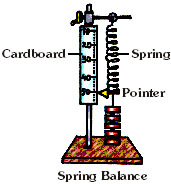NOTE :– Spring applies force on both ends. These forces are equal in magnitude but opposite in direction.
A spring balance consists of a spring inserted in a metal tube. The metal tube has a vertical slop through which the pointer comes out. The object to be weight is
suspended on the hook at the bottom. When the object comes to equilibrium it stretches the spring. As a result, the pointer comes down and shows the reading against a scale.

PRESSURE
It is defined as the force per unit area. The SI unit of pressure is Pascal (Pa), which is Newton per square metre.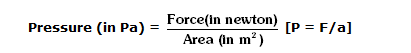Ex.    If a force of 2 N is applied over an area of 2 cm2, calculate the pressure produced.
Sol.    To get the pressure in Pa, we have to make sure that the force is in Newton and the area in m2.
Here, the area is in cm2. To convert this to m2, we have to divide the given are a by 10, 000
Thus, area    = 2/10,000  = 0.0002 m2
Now, Pressure = FORCE/AREA = 2N/0.0002m2 = 10, 000 Pa
Ex.    Calculate the pressure if a force of 2N is applied on an area of 2 mm2. Here, again the area is not in m2. To change it into m2, we divide the area by 1, 000,000.
Sol.     Thus, area    = 2/1,000,000  = 0.000002 m2
Now, Pressure =  FORCE/AREA= 2N/0.000002m2 = 1,000,000 Pa

VARIATIONS OF PRESSURE WITH AREA
The same force, increasing the area over which it acts decreases the pressure applied. The inverse is also true: decreasing the area of application increases the pressure produced for the same force.
Ex. The area under the edge of a knife's blade is extremely small.
Beneath it the pressure is high enough for the blade to push
easily through the material that needs to be cut.

Wall foundations have a large horizontal area. This reduces the
pressure underneath so that the walls do not sink further into the
ground under the weight of the building.

UNITS OF PRESSURE

The SI unit of pressure is called Pascal (Pa) in honour of Blaise Pascal.
I Pa = 1 N/m2
One Pascal is defined as the pressure exerted on a surface area of 1 m2 by a thrust of 1 N (acting normally on it).
Other units:
(i)  In C.G.S unit of pressure is dyne/cm2
(ii)  1 bar = 105 N/m2
(iii) 1 milibar = 102 N/m2
(iv)  1 atmospheric pressure (1 atm) = 101·3 k Pa = 1·013 bar = 1013 m bar = 760 mm of Hg
(v)   1 Torr = 1mm of Hg

PRESSURE IN FLUIDS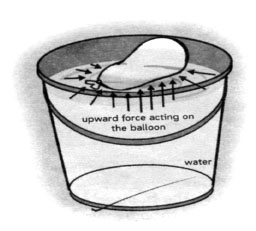Liquids and gases are together called fluids.
Fluids exert pressure on all bodies immersed in them and on the walls of  the container that holds them. The air inside the balloon exerts a pressure on the inner walls of the balloon.

LAWS OF PRESSURE

(i)    Pressure exerted by the liquid is the same in all directions about a points
(ii)     Pressure exerted is the same at all points in a horizontal plane as well as in a stationary liquid.
(iii)    Pressure at a points inside a liquid increases with depth from the free surface.
(iv)     Pressure at a particular depth is different for different liquids, i.e. P = hdg
where,    h = height of the column of liquid.    d = density of the liquid
g = acceleration due to gravity
(v)     The pressure exerted anywhere in a confined liquid is transmitted equally and undiminished in all directions throughout the liquid which is called 'Pascal's law'.

PASCAL'S LAW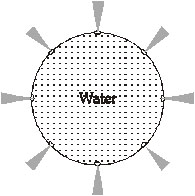In an enclosed fluid, if pressure is changed in any part of the fluid, then this change of pressure is transmitted undiminished to all the other parts of the fluid.

ATMOSPHERIC PRESSURE
A layer of air called the atmosphere surrounds the earth. As you know, air is also matter and has weight. The weight of the atmosphere exerts a pressure on the
surface of earth. This pressure is called atmospheric pressure. Its magnitude is around 100 kilo pascals (100 kPa) at sea level. However, as we go upward, the
magnitude of atmospheric pressure decreases gradually. The following activity will show the magnitude of atmospheric pressure on the earth's surface.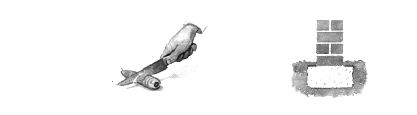THE MAGNITUDE OF ATMOSPHERIC PRESSURE
Take a glass tumbler and fill it with water to the brim. Cover it with a thick sheet of paper (or cardboard). Press your palm over the sheet and quickly
invert the tumbler. Slowly remove your palm supporting the piece of paper. What do you observe? Surprised?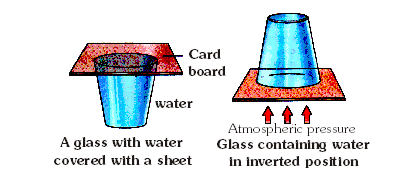You have seen that the paper did not fall (as one expected it to.) This is because the atmospheric pressure provides enough force to push the piece of paper upward.

BAROMETER
A barometer is a device used for measuring the atmospheric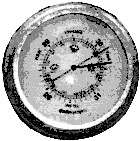pressure. Atmospheric pressure measurements are important to meteorologists

for weather forecasts. The unit of pressure used for meteorological purpose is called the bar.

A bar is a CGS unit of pressure and equals 105 Pascals or 1 bar = 105 Nm–2.

LIQUID PRESSURE
The normal force (or thrust) exerted by a liquid at rest per unit area of the surface in contact with it is called "pressure of liquid or hydrostaticpressure."Take some discarded plastic bottle and fix a glass tube near its bottom. It can be done by slightly heating one end of the glass tube and then inserting

it near the bottom of the bottle. In case there is some leakage, your must seal it with molten wax. Now cover the free end of the glass tube with a thin rubber sheet. On
filling the plastic bottle upto half with water, the rubber sheet fixed to the glass tube bulges. When more water is added in the plastic bottle, there is change in the bulge of the rubber sheet. Since the rubber sheet is fixed on the side of the container, it shows that water exerts pressure on the side of the container. In other words, liquids exert pressure on the walls of the container.

MANOMETER

It is an a instrument used to measure liquid pressure. An open tube manometer is a simplest type of pressure gauge which measures pressure. It consists of a
U-shaped tube containing a liquid. One arm of the tube is open to air and the other arm is connected to the vessel in which we want to measure the pressure. The difference
in liquid level represents the applied pressure.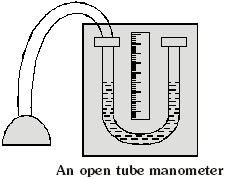OTHERS APPLICATIONS OF PRESSURE
(a) Drinking straw  Straw is used to suck up aerated water, when air is sucked in, it causes a decrease in air pressure inside the straw. The outside atmospheric
pressure forces the liquid inside the straw. The dropper also works on the same principle. This is known as suction mechanism.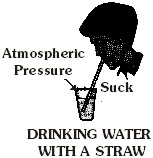(b) Syringe   In syringe the pressure of the liquid (blood) force the liquid
to move into the syringe when its plunger is withdrawn.(c) Vacuum cleaner  A vacuum cleaner is an electrical appliance that cleans by suction. A fan inside the vacuum cleaner lower the air pressure and creates a low
pressure the device. As a result, the air and dirt particles on and near the surface are sucked into the device.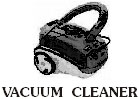(d) Sphygmomanometer An instrument called sphygmomanometer is used to measure the blood pressure of humans. The blood pressure of a person
is the ratio of systolic (maximum) and diastolic (minimum) pressures. Normally it is 120/80 mm of Hg in a healthy adult. Pressures above 140/90 needs medical attention.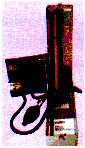DENSITY

Density of a substance is defined as its mass per unit volume.
density(d) =
Unit of density :- Since mass (M) is measured in kilogram (kg) and the volume (V) is measured in metre3 (m3), the unit of density is kg/m3. In cgs system, the unit of density is g cm–3.
These units are related as : 1 g cm–3 = 1000 kgm–3.

BUOYANCY

When a body is partially or wholly immersed in a liquid, an upward force acts on it which is called upthrust or buoyant force. The property of the liquids responsible
for this force is called buoyancy.
Buoyancy is a familiar phenomenon : a body immersed in water seems to weigh less than when it is in air. When the body is less dense than the fluid, then it floats.
The human body usually floats in water, and a helium filled balloon floats in air.
When a body is immersed in a fluid (liquid or gas) it exerts an upward force on the body. This upward force is called upthrust or buoyant force (U or FB) and the
phenomenon is termed as buoyancy. Thus buoyancy or upthrust is the upward force exerted by a fluid (liquid or gas) when a body is immersed in it.
It is a common experience that when a piece of cork is placed in water it floats with two-fifth of its volume inside water. If the cork piece is pushed into water and
released it comes to the surface as if it has been pushed by someone from inside due to the buoyant force exerted by fluid.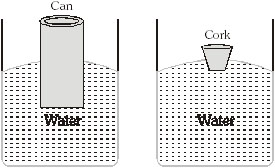ARCHIMEDES PRINCIPLE

Archimedes principle states that :- "Anybody completely or partially submerged in a fluid is buoyant up by a force equal to the weight of the fluid displaced by the liquid".
In other words :- "When a body is partially or completely immersed in a fluid, the fluid exerts an upward force on the body equal to the weight of the fluid displaced by the body.

LAW OF FLOATATION

Law of floatation is an extension of Archimedes principle.
When a body is partially or fully immersed in a fluid, then following vertical forces are experienced by it.
(a)    Its weight (W) acting vertically downwards through the centre of the body.
(b)    Force of buoyancy (B or FB) or upthrust, acting vertically upwards through the centre of gravity of the body.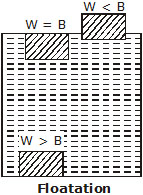The following three cases arise :-
(1) When W < B, the body floats : In this case the body will rise above the surface on the liquid to the extent that the weight of the liquid displaced by its
immersed part equals the weight of the body. Then the body will float with only a part of it immersed in the liquid. In this case Vrg < Vsg or r < s. Thus if a
cork, which has a density less than that of water will rise in water till a portion of it is above water. Similarly a ship floats in water since its density is less than the density of water.

(2) When W > B, the body sinks :- If r and s present the densities of the body and the fluid respectively and V the volume of the body (which is also the volume of the fluid displaced)
then Vrg > Vsg or r > s i.e., the body sinks in the fluid in case its density is greater than the density of the fluid. An iron nail has greater density than water, therefore it sinks in water.

(3) When W = B :- The resultant force acting on the body when fully immersed in the fluid is zero. The body is at rest anywhere within the fluid.
The apparent weight of the body is zero for all such positions.
Thus, we find that a body will float when its weight is equal to the weight B of the fluid displaced i.e. the upthrust.

SOLVED EXAMPLES

Ex.1    In a tug of war, the three members of team A pull with a force of 100 N, 120 N, and 135N. The three members of team B pull with a force
of 130 N, 105 N, and 120 N which team will win?
Sol.    Force exerted by team A = 100 N + 120 N + 135 N = 355 N.
Force exerted by team B = 130 N + 105 N + 120 N = 355 N.
Teams are pulling with same force
The resultant force is zero. Neither team will win. (Ans.)

Ex.2    A force of 20 N acts over on area of 4 m2. What is the pressure?
Sol.    Given : F = 20 N, A = 4 m2, pressure?
We know that pressure = =  = 5 N/m2 or 5 Pa.

Ex.3    A pressure of 50 N/m2 acts on an area of 5 m2. Calculate the total force.
Sol.    Given : P = 50 N/m2, A = 5 m2, force?
We know that pressure =
Force = Area × pressure = 5m2 × 50 N/m2
F = 250 N (Ans.)

Ex.4    A force of 800 N exerts a pressure of 40 N/m2. What are is it action?
Sol.    Given : F = 800 N, P =40 N/m2, Area?
We know that pressure =
\    = Area = force/pressure =  = 20 m2

Ex.5    A person weigh 600 N. He is wearing shoes with a total area of 0.02 m2. What pressure do they exert on the floor?
Sol.    Given : F = 600 N, A = 0.02 m2, pressure?
We know that pressure = =  = 30000 N/m2 or 30 KPa. (Ans.)

Ex.6    In a game of tug of war, three girls of team A pull the rope with forces of 100 N, 120 N and 170 N. In team B, the three members pull the rope with
force of 130 N, 150 N and 155 N. Who will win the rug of war? What is the resultant force?
Sol.    [Team A will win, 45 N]

Ex.7    A horse pulls a cart with a force of 1500 N. The force of friction between the cart and the ground is 1500 N. The cart does not move. Why?
Sol.    [Same force applied in opposite direction so resultant force zero]

Ex.8    Define pressure. What are the units of pressure in this list: N/cm2, N, N/m2, cm2, m2
Sol.    [N/m2 or N/cm2]

Ex.9    A force of 500 N acts on a square piece of plywood, each of whose sides is 5 m long. Calculate the pressure acting on the piece of plywood.
Sol.    [20 N]

Ex.10    A body stands on the ground. The area below his feet is 70 cm2. The pressure he exerts on the ground is 7 N/cm2. Calculate the total force acting on the ground.
Sol.    [490 N/cm2]

Ex.11    A force exerts a pressure of 45 N/m2 when it acts on an area of 10 m2. Calculate the total force.
Sol.    [450 N/m2]

Ex.12    A force of 400 N exerts pressure of 20 N/cm2. What is the area on which the force acts?
Sol.    [20 N]

Ex.13    The picture shows a heavy box placed on the floor in three different ways. In which case would the pressure on the floor be the least? When would it be the most? Why?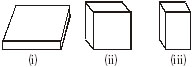Sol.    [Fig (iii) least pressure because is area is minimum]

Ex.14    You want to lift a heavy box. The force of gravity pulls it down wards with a force of 500 N. Your father applies an upward force of 220 N form below.
how much force will you have to apply to lift it upwards?
Sol.    [Less than 220 N]

Ex.15    The surface area of the end of a brick 50 cm2. The surface area of the base brick is 200 cm2. Each brick weighs 50 N. What pressure is exerted on the
ground by the brick in the two cases shown here?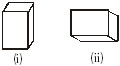Sol.    [(i) for standing brick 1N/cm2
[(ii) For the brick lying on its box = 0.25 N/cm2]

NCERT QUESTIONS WITH SOLUTIONS

Q.1    Give two examples of each of situation in which you push or pull to change the state of motion of an objects
Ans.    (i) We push a bicycle to move it
(ii) We pull the table to change its position.
Q.2    Give two examples of situations in which applied force causes a change in the shape of an object.
Ans.    (i) When we press the foam, its shape is changed.
(ii) When we stretch the rubber bend, then its shape is changed.
Q.3    Fill in the blanks
(a) To draw water from a well we have to ______ at rope.
(b) A charged body _____  an uncharged body towards it.
(c) To move a loaded trolley we have to ______ it.
(d) The north pole of a magnet ______ the north pole of another magnet.
Ans.    (a) Pull             (b) Attracts            (c) Push        (d) Repels
Q.4    An archer stretches her bow while taking aim at the target. She then releases the arrow, which begins to move towards the target. Based on this information
fill up the gaps in the following statements using the following terms :
muscular, contact, non-contact, gravity, friction, shape, attraction
(a) To stretch the bow, the archer applies a force that causes a change in its _____ .
(b) The force applied by the archer to stretch the bow is an example of ______ force.
(c) The type of force responsible for a change in the state of motion of the arrow is an example of  a _______ force.
(d) While the arrow moves towards its target, the forces acting on it are due to _______ and that due to _______ of air.
Ans.    (a) shape        (b) muscular            (c) contact        (d) gravity, friction
Q.5    In the following situations identify the agent exerting a force and the object on which it acts. State the effects of the force in each case.
(a) Squeezing a piece of lemon between the fingers to extract its juice.
(b) Taking out paste from a toothpaste tube.
(c) A load suspended from a spring while its other end is on a hook fixed to a wall.
(d) An athlete making a high jump to dear the bar at a certain height.
Ans.    (a) The fingers are the agents, lemon is the object.
The effect of force is the lemon juice being expelled by squeezing.
(b) The hand is the agent, toothpaste tube is object and the coming out of paste from toothpaste tube is the effect of force.
(c) Suspended load is agent, spring is the object, the effect of force can be seen in the form of elongation of spring on suspension of load.
(d) Athlete is the agent, bar is the object. The force can be seen In the form of jump.
Q.6    A blacksmith hammers a hot piece of iron while making a tool. How does the force due to hammering affect the piece of iron ?
Ans.    The force due to hammering causes the change in shape of iron and iron can be moulded in the shape of the required tool.
Q.7    An inflated balloon was pressed against a wall after it has been rubbed with a piece of synthetic cloth. It was found that the balloon sticks to the wall.
What force might be responsible for the attraction between the balloon and the wall ?
Ans.    Electrostatic force.
Q.8    Name the force acting on a plastic bucket containing water held above ground level in your hand. Discuss why the forces acting on the bucket do not
bring a change in its state of motion.
Ans.    Muscular and gravitational forces act on plastic bucket. The force acting on the bucket do not bring a change in state of motion because they are acting in
opposite direction with equal magnitudes.
Therefore the net force on bucket remains zero.
Q.9    A rocket has been fired upward to launch a satellite in its orbit. Name the two forces acting on the rocket immediately after leaving the launching pad.
Ans.    (i) Gravitational force                 (ii) Force of friction.
Q.10    When we press the bulb of a dropper with its nozzle kept in water, air in the dropper is seen to escape in the form of bubbles. Once we release the pressure
on the bulb, water gets filled in dropper. The rise of water in the dropper is due to
(a) pressure of water                (b) gravity of the earth
(c) shape of rubber bulb            (d) atmospheric pressure
Ans.    (d) atmospheric pressure.

EXERCISE - I

Q.1    Is weight a force? Write the SI unit of force.
Q.2    What are different types of forces?
Q.3    Define 1 kgf.
Q.4    Name two units of force.
Q.5    Is force a scalar or a vector?
Q.6    What are electrostatic forces?
Q.7    What is the main use of a spring balance?
Q.8    Write the SI unit of pressure.
Q.9    What do you mean by thrust?
Q.10    What is a manometer?
Q.11    What is atmospheric pressure at sea level?
Q.12    Distinguish between mass and weight.
Q.13    Define weight of a body. Name the unit used to measure it.
Q.14    State and explain Pascal's law. Does it apply to gases also?
Q.15    What are the factors on which the pressure of a liquid depends?
Q.16    Why are railway tracks laid on wooden or iron sleepers?
Q.17    Why do we feel pain when we walk on a ground having small pebbles?
Q.18    Explain how it is possible to drink a liquid by using a straw?
Q.19    Why are dams made broader at the bottom than at the top?
Q.20    Explain in suitable detail, the effect of force on a body.
Q.21    Explain the basic principle of gravitational force. How will you measure it? Write its SI unit.
Q.22    Define force. Briefly explain different units of force.
Q.23    (i) What do you mean by atmospheric pressure?
(ii) Why does a fountain pen start leaking at higher altitudes?
Q.24    Briefly explain the principle, construction and working of a manometer.
Q.25    (i) State Pascal's law
(ii) Give two examples of pressure in everyday life.
Q.26    Why is one end of a drawing pin kept wide, but the other end very sharp?
Q.27    What is force? Explain the four effects a force can produce, giving relevant examples.
Q.28    Explain contact and non-contact forces by
giving suitable examples.
Q.29    Distinguish between thrust and pressure. Write their units. What is the relation between them?

EXERCISE - II

Q.1    If a rock is brought from the surface of the moon :
(A) Its mass will change
(B) Its weight will change, but not mass.
(C) Both mass and weight will change    (D) Its mass and weight will remain the same.
Q.2    When an object undergoes acceleration :
(A) Its speed always increases
(B) Its velocity always increases
(C) It always falls towards the earth    (D) A force always acts on it
Q.3    External forces are :
(A) Always balanced
(B) Never balanced
(C) May or may not be balanced
(D) None of these
Q.4    The net force acting on a body of mass 1 kg moving with a uniform velocity of 5 ms–1 is :
(A) 5 N    (B) 0.2 N
(C) 0 N    (D) None of these
Q.5    How many dynes are equal to 1 N?
(A) 106    (B) 104
(C) 105    (D) 103
Q.6    A force can :
(A) Change the direction of a moving body    (B) Change the state of rest or uniform motion of a body.
(C)  Change the shape of a body
(D) All of the above
Q.7    The S.I. unit of pressure is –
(A) Newton    (B) Dyne/cm2
(C) Pascal    (D) Joule
Q.8    Which among the following will exert maximum pressure when pushed with the same amount of force?
(A) An eraser of area 2 cm2
(B) A sharpened pencil tip
(C) The blunt end of a pencil
(D) The rear portion of a closed safety pin
Q.9    Pressure is also measured in :
(A) Joule    (B) mm of Hg
(C) mm of Ag    (D) Metre
Q.10    Force per unit area is called :
(A) Energy     (B) Work
(C) Pressure    (D) Thrust
Q.11    Atmospheric pressure is measured by :
(A) Barometer    (B) Manometer    (C) Screw gauge    (D) None of these
Q.12    A manometer is used to measure :
(A) Height
(B) Pressure
(C) Liquid density
(D) Atmospheric pressure
Q.13    How does pressure vary as we come from mountain top to sea level?
(A) Increases    (B) Decreases
(C) Remains same    (D) Depends on weather
Q.14    As we go deeper beneath the surface of liquid, the pressure :
(A) Remains same    (B) Increase
(C) Decreases    (D) Depends on weather
Q.15    A vacuum cleaner works on the principle of :
(A) Electromagnetic Induction
(B) Suction
(C)  Mutual Induction
(D) Energy conservation
Q.16    The S.I. unit of force is :
(A) metre    (B) Newton
(C) Pascal    (D) Second
Q.17    A contact force cannot act through
(A) Empty space
(B) Touching
(C) Touching with a metal rod
(D) Touching with a wooden rod
Q.18    Which of the following is a unit of pressure?
(A) Metre     (B) Newton
(C) Square metre    (D) Pascal
Q.19    Deep-sea diving vessels have to withstand pressure from the crushing effect of sea water acting :
(A) Upwards    (B) Downwards    (C) Side ways    (D) In all directions.
Q.20    Which of the following is a type of a pressure gauge?
(A) Speedometer    (B) Manometer    (C) Syringe    (D) Thermometer
Q.21    Which of these is not a contact force?
(A) Friction    (B) Muscular force    (C) Magnetic force    (D) None of these
Q.22    A force has :
(A) Magnitude only
(B) Direction only
(C) both magnitude and direction
(D) None of these
Q.23    When a force is applied on a body it may change its
(A) Speed only
(B) Direction only
(C) both speed and direction
(D) None of these
Q.24    When we press the bulb of a dropper with its nozzle kept in water, air in the dropper is seen to escape in the form of bubbles. Once we release the pressure
on the bulb, water gets filled in the dropper. The rise of water in the dropper is due to :
(A) Pressure of water
(B) Gravity of the earth
(C) Shape of rubber bulb
(D) Atmospheric pressure
Q.25    Gravitational force acts :
(A) Only between the sum and the planets moving around it.
(B) Only between the earth and the bodies on it
(C) Between all bodies in the universe
(D) Only between the sun and the earth
Q.26    The relation between the S.I. unit of force and the weight of a 1 kg mass is :
(A) 1 kgf = 1 N    (B) 1 kgf = 0.98 N    (C) 1 kgf = 9.8 N    (D) 1 N = 9.8 kg
Q.27    If a force of 100 N acts on an area of 10 m2, the pressure equal :
(A) 100 N/m2    (B) 10 N/m2
(C) 1000 N/m2    (D) 1000 Nm2
Q.28    1 dyne is equal to :
(A) 980 g wt    (B) 1/980 g wt    (C) 980 kg wt    (D) None of these
Q.29    Which of the following class of force is different from others?
(A) magnetic force
(B) Electrical force
(C) Gravitational force
(D) Stretching of a spring

1.    B    2.    D    3.    C    4.    C
5.    C    6.    D    7.    C    8.    B
9.    B    10.    C    11.    A    12.    B
13.    A    14.    B    15.    B    16.    B
17.    A    18.    D    19.    D    20.    B
21.    C    22.    C    23.    C    24.    D
25.    C    26.    C    27.    A    28.    B
29.    D

EXERCISE - III

1.    If a rock is brought from the surface of the moon :
(A)    Its mass will change
(B) Its weight will change, but not mass.
(C)    Both mass and weight will change    (D) Its mass and weight will remain the same.
2.    When an object undergoes acceleration :
(A)    Its speed always increases
(B) Its velocity always increases
(C)    It always falls towards the earth    (D) A force always acts on it
3.    External forces are :
(A)    Always balanced    (B) Never balanced
(C) May or may not be balanced    (D) None of these
4.    The net force acting on a body of mass 1 kg moving with a uniform velocity of 5 ms–1 is :
(A)    5 N    (B) 0.2 N
(C) 0 N    (D) None of these
5.    How many dynes are equal to 1 N?
(A)    106    (B) 104
(C) 105    (D) 103
6.    A force can :
(A)    Change the direction of a moving body    (B) Change the state of rest or uniform motion of a body.
(C)     Change the shape of a body    (D) All of the above
7.    The S.I. unit of pressure is –
(A)    Newton    (B) Dyne/cm2    (C) Pascal    (D) Joule
8.    Which among the following will exert maximum pressure when pushed with the same amount of force?
(A)    An eraser of area 2 cm2    (B) A sharpened pencil tip
(C)    The blunt end of a pencil    (D) The rear portion of a closed safety pin
9.    Pressure is also measured in :
(A)    Joule    (B) mm of Hg    (C) mm of Ag    (D) Metre
10.    Force per unit area is called :
(A)    Energy     (B) Work     (C) Pressure    (D) Thrust
11.    Atmospheric pressure is measured by :
(A)    Barometer    (B) Manometer    (C) Screw gauge    (D) None of these
12.    A manometer is used to measure :
(A)    Height    (B) Pressure    (C) Liquid density    (D) Atmospheric pressure
13.    How does pressure vary as we come from mountain top to sea level?
(A)    Increases    (B) Decreases    (C) Remains same    (D) Depends on weather
14.    As we go deeper beneath the surface of liquid, the pressure :
(A)    Remains same    (B) Increase    (C) Decreases    (D) Depends on weather
15.    A vacuum cleaner works on the principle of :
(A)    Electromagnetic Induction    (B) Suction
(C)     Mutal Induction         (D) Energy conservation
16.    The S.I. unit of force is :
(A)    metre    (B) Newton    (C) Pascal    (D) Second
17.    A contact force cannot act through
(A)    Empty space        (B) Touching
(C) Touching with a metal rod    (D) Touching with a wooden rod
18.    Which of the following is a unit of pressure?
(A)    Metre     (B) Newton    (C) Square metre    (D) Pascal
19.    Deep-sea diving vessels have to withstand pressure from the crushing effect of sea water acting :
(A)    Upwards    (B) Downwards    (C) Side ways    (D) In all directions.
20.    Which among the following will exert maximum pressure when pushed with the same amount of force?
(A)    An eraser of area 2 cm2    (B) A sharpened pencil tip
(C)     The blunt end of a pencil    (D) The rear portion of a closes safety pin.
21.    Which of the following is a type of a pressure gauge?
(A)    Speedometer    (B) Manometer    (C) Syringe    (D) Thermometer
22.    Which of these is not a contact force?
(A)    Friction    (B) Muscular force    (C) Magnetic force    (D) None of these
23.    A force has :
(A)    Magnitude only    (B) Direction only    (C) both magnitude and direction    (D) None of these
24.    When a force is applied on a body it may change its
(A)    Speed only    (B) Direction only    (C) both speed and direction    (D) None of these
25.    When we press the bulb of a dropper with its nozzle kept in water, air in the dropper is seen to escape in the form of bubbles. Once we release the pressure on the
bulb, water gets filled in the dropper. The rise of water in the dropper is due to :
(A)    Pressure of water    (B) Gravity of the earth    (C) Shape of rubber bulb    (D) Atmospheric pressure
26.    Gravitational force acts :
(A)    Only between the sum and the planets moving around it.
(B)     Only between the earth and the bodies on it
(C)     Between all bodies in the universe
(D)    Only between the sun and the earth
27.    The relation between the S.I. unit of force and the weight of a 1 kg mass is :
(A)    1 kg of = 1 N    (B) 1 kg of = 0.98 N    (C) 1 kg of = 9.8 N    (D) 1 N = 9.8 kg
28.    If a force of 100 N acts on an area of 10 m2, the pressure equal :
(A)    100 N/m2    (B) 10 N/m2    (C) 1000 N/m2    (D) 1000 Nm2
29.    1 dyne is equal to :
(A)    980 g wt    (B) 1/980 g wt    (C) 980 kg wt    (D) None of these
30.    Which of the following class of force is different from others?
(A)    magnetic force    (B) Electrical force    (C) Gravitational force    (D) Stretching of a spring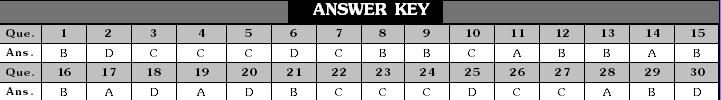Fill in the blanks
31.    The ............. or push acting on a body is commonly called force
32.    The force of gravity is a ............. force.
33.    Hydraulic press is based on .............
34.    To draw water from a well we have to ............. at the rope.
35.    A charged body ............. an uncharged body towards it.
36.    To move a loaded trolley we have to ............. it.
37.    The north pole of a magnet ............. the north pole of another magnet.
38.    To stretch the how, the archer applies a force that causes a change in its .............
39.    The force applied by the archer to starch the bow is an example of ............. force.
40.    A manometer is used to measure .............
41.    Pascal (Pa) is the unit of  .............
42.    ............. and ............. are together called fluids.
43.    An instrument used to measure ............. is called a pressure gauge.
44.    Pressure is also measured in .............
45.    Hydraulic devices are based on .............
Complete the following table
Pressure       Force      Area
46.    _________        50 N        5 cm2
47.    15N/cm2    ___________        5 cm2
48.    5N/m2        500 N      ___________
49.    _________        750 N        25 cm2
50.    80 N/m2        800 N      ___________
51.    45 N/m2    ___________        10 m2
52.    _________        200 N        10 cm2
53.    5N/m2    ___________        20 m2
54.    20 N/m2        400 N      ___________
55.    40 N/m2        800 N      ___________

Match the Column
56.           Column-A                     Column-B
(A)    Opening a draw                (i)    Push
(B)    Electrostatic force            (ii)     Contact force
(C)    Moving a book placed on a table        (iii)Pull
(D)    Muscular force                (iv)Push or pull
(E)    Force                (v)     Non-contact force
(F)    Magnetic force                (vi) Non-contact force
(G)    A physical quantity that determines        (vii) Depth
the pressure in liquids.
(H)    SI unit of force                (viii)Friction
(I)    Spring balance                (ix) Muscular force.
(J)    Force opposing the motion        (x)     Weight
(K)    Force exerted by muscles        (xi) Newton
(L)    Thrust (SI unit)                (xii) kg wt
(M)Hydrostatic pressure            (xiii) N
(N)    Weight                (xiv) Nm–2

Give reasons for the following
57.    Skis have a large area of contract with snow.
58.    A needle has a pointy tip.
59.    It is easier to use a sharp knife as compared to a blunt one.
60.    Spaceman have to wear special pressurized suits.
61.    Dams are made stronger and thicker at the bottom that at the top.
62.    Deep sea divers have to wear specially designed suits.
63.    Building foundations have a large horizontal area of contact with the ground.
64.    On the basis of interaction what are two categories of force.
65.    Name some non-contact forces.
66.    Does liquids also exert pressure.
67.    Athletes wear specially designed shoes with spikes on the soles.
68.    If you walk on the show, yourself will sink, but if you put on skis you can move over the snow easily.
69.    A woman walking across a lawn in high-heeled shoes would leave a deeper impression on the ground than an elephant.
70.    It is easier to sew with a pointed needle than with a blunt needle.
71.    It is necessary to keep the bathroom floor clean and  free of oily substances.

Fill in the blanks
31.    Pull    32.    Gravitational
33.    Gravity    34.    Downward
35.    Attarct    36.    Pull
37.    Repal    38.    Position
39.    Contact    40.    Pressure difference
41.    Pressure    42.    Gas, Liquids
43.    Push or pull    44.    pushes
45.    Pascal's Law